## In April 1974, Steve Prefontaine completed a 10.0 km10.0 km race in a time of 27 min27 min , 43.6 s43.6 s . Suppose “Pre” was at the 7.85 km

Question

In April 1974, Steve Prefontaine completed a 10.0 km10.0 km race in a time of 27 min27 min , 43.6 s43.6 s . Suppose “Pre” was at the 7.85 km7.85 km mark at a time of 25.0 min25.0 min . If he accelerated for 60 s60 s and then maintained his increased speed for the remainder of the race, calculate his acceleration over the 60 s60 s interval. Assume his instantaneous speed at the 7.85 km7.85 km mark was the same as his overall average speed up to that time.

in progress 0
3 months 2021-07-25T17:17:46+00:00 1 Answers 6 views 0

a = 0.161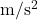Explanation:

Given :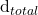= 10 km = 10000 m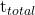= 27 min 43.6 s

= 1663.6 s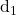= 7.85 km = 7850 m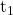= 25 min = 1500 s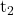= 60 s

Now the initial speed for the distance of 7.85 km is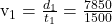= 5.23 m/s

The velocity after 60 s after the distance of 7.85 kn is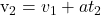= 5.23 + a(60)

The distance traveled for 60 s after the distance of 7.85 km is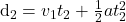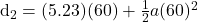= 313.8 + a(1800)

The time taken for the last journey where the speed is again uniform is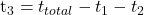= 1663.6 – 1500 – 60

= 103.6 s

Therefore, the distance traveled for the time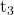is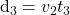= (5.23+60a)(103.6)

= 541.8 + 6216 a

The total distance traveled,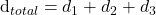Now substituting the values in the above equation for the acceleration a is

10000 = 7850 + (313.6 + 1800a) + (541.8 + 6216a)

10000 = 8706.5 + 8016a

1294.4 = 8016a

a = 0.161